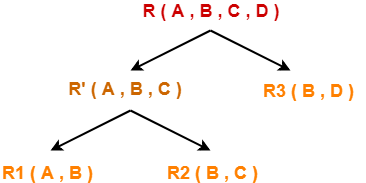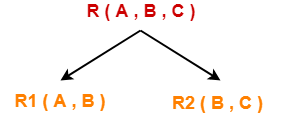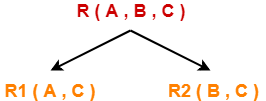## Decomposition in DBMS-

Before you go through this article, make sure that you have gone through the previous article on Decomposition in DBMS.

We have discussed-

• Decomposition is a process of dividing a single relation into two or more sub relations.
• Decomposition of a relation can be completed in the following two ways-1. Lossless Join Decomposition
2. Lossy Join Decomposition

In this article, we will learn how to determine whether the decomposition is lossless or lossy.

## Determining Whether Decomposition Is Lossless Or Lossy-

Consider a relation R is decomposed into two sub relations R1 and R2.

Then,

• If all the following conditions satisfy, then the decomposition is lossless.
• If any of these conditions fail, then the decomposition is lossy.

## Condition-01:

Union of both the sub relations must contain all the attributes that are present in the original relation R.

Thus,

 R1 ∪ R2 = R

## Condition-02:

• Intersection of both the sub relations must not be null.
• In other words, there must be some common attribute which is present in both the sub relations.

Thus,

 R1 ∩ R2 ≠ ∅

## Condition-03:

Intersection of both the sub relations must be a super key of either R1 or R2 or both.

Thus,

 R1 ∩ R2 = Super key of R1 or R2

## Problem-01:

Consider a relation schema R ( A , B , C , D ) with the functional dependencies A → B and C → D. Determine whether the decomposition of R into R1 ( A , B ) and R2 ( C , D ) is lossless or lossy.

## Solution-

To determine whether the decomposition is lossless or lossy,

• We will check all the conditions one by one.
• If any of the conditions fail, then the decomposition is lossy otherwise lossless.

## Condition-01:

According to condition-01, union of both the sub relations must contain all the attributes of relation R.

So, we have-

R1 ( A , B ) ∪ R2 ( C , D )

= R ( A , B , C , D )

Clearly, union of the sub relations contain all the attributes of relation R.

Thus, condition-01 satisfies.

## Condition-02:

According to condition-02, intersection of both the sub relations must not be null.

So, we have-

R1 ( A , B ) ∩ R2 ( C , D )

= Φ

Clearly, intersection of the sub relations is null.

So, condition-02 fails.

Thus, we conclude that the decomposition is lossy.

## Problem-02:

Consider a relation schema R ( A , B , C , D ) with the following functional dependencies-

A → B

B → C

C → D

D → B

Determine whether the decomposition of R into R( A , B ) , R2 ( B , C ) and R3 ( B , D ) is lossless or lossy.

## Solution-

### Strategy to Solve

When a given relation is decomposed into more than two sub relations, then-

• Consider any one possible ways in which the relation might have been decomposed into those sub relations.
• First, divide the given relation into two sub relations.
• Then, divide the sub relations according to the sub relations given in the question.

As a thumb rule, remember-

Any relation can be decomposed only into two sub relations at a time.

Consider the original relation R was decomposed into the given sub relations as shown-## Decomposition of R(A, B, C, D) into R'(A, B, C) and R3(B, D)-

To determine whether the decomposition is lossless or lossy,

• We will check all the conditions one by one.
• If any of the conditions fail, then the decomposition is lossy otherwise lossless.

## Condition-01:

According to condition-01, union of both the sub relations must contain all the attributes of relation R.

So, we have-

R ( A , B , C ) ∪ R3 ( B , D )

= R ( A , B , C , D )

Clearly, union of the sub relations contain all the attributes of relation R.

Thus, condition-01 satisfies.

## Condition-02:

According to condition-02, intersection of both the sub relations must not be null.

So, we have-

R ( A , B , C ) ∩ R3 ( B , D )

= B

Clearly, intersection of the sub relations is not null.

Thus, condition-02 satisfies.

## Condition-03:

According to condition-03, intersection of both the sub relations must be the super key of one of the two sub relations or both.

So, we have-

R ( A , B , C ) ∩ R3 ( B , D )

= B

Now, the closure of attribute B is-

B+ = { B , C , D }

Now, we see-

• Attribute ‘B’ can not determine attribute ‘A’ of sub relation R’.
• Thus, it is not a super key of the sub relation R’.
• Attribute ‘B’ can determine all the attributes of sub relation R3.
• Thus, it is a super key of the sub relation R3.

Clearly, intersection of the sub relations is a super key of one of the sub relations.

So, condition-03 satisfies.

Thus, we conclude that the decomposition is lossless.

## Decomposition of R'(A, B, C) into R1(A, B) and R2(B, C)-

To determine whether the decomposition is lossless or lossy,

• We will check all the conditions one by one.
• If any of the conditions fail, then the decomposition is lossy otherwise lossless.

## Condition-01:

According to condition-01, union of both the sub relations must contain all the attributes of relation R’.

So, we have-

R( A , B ) ∪ R2 ( B , C )

= R’ ( A , B , C )

Clearly, union of the sub relations contain all the attributes of relation R’.

Thus, condition-01 satisfies.

### Condition-02:

According to condition-02, intersection of both the sub relations must not be null.

So, we have-

R1 ( A , B ) ∩ R2 ( B , C )

= B

Clearly, intersection of the sub relations is not null.

Thus, condition-02 satisfies.

## Condition-03:

According to condition-03, intersection of both the sub relations must be the super key of one of the two sub relations or both.

So, we have-

R1 ( A , B ) ∩ R2 ( B , C )

= B

Now, the closure of attribute B is-

B+ = { B , C , D }

Now, we see-

• Attribute ‘B’ can not determine attribute ‘A’ of sub relation R1.
• Thus, it is not a super key of the sub relation R1.
• Attribute ‘B’ can determine all the attributes of sub relation R2.
• Thus, it is a super key of the sub relation R2.

Clearly, intersection of the sub relations is a super key of one of the sub relations.

So, condition-03 satisfies.

Thus, we conclude that the decomposition is lossless.

### Conclusion-

Overall decomposition of relation R into sub relations R1, R2 and R3 is lossless.

Next Article- Introduction to Normal Forms

Get more notes and other study material of Database Management System (DBMS).

Watch video lectures by visiting our YouTube channel LearnVidFun.

## Decomposition of a Relation-

 The process of breaking up or dividing a single relation into two or more sub relations is called as decomposition of a relation.

## Properties of Decomposition-

The following two properties must be followed when decomposing a given relation-

## 1. Lossless decomposition-

Lossless decomposition ensures-

• No information is lost from the original relation during decomposition.
• When the sub relations are joined back, the same relation is obtained that was decomposed.

Every decomposition must always be lossless.

## 2. Dependency Preservation-

Dependency preservation ensures-

• None of the functional dependencies that holds on the original relation are lost.
• The sub relations still hold or satisfy the functional dependencies of the original relation.

## Types of Decomposition-

Decomposition of a relation can be completed in the following two ways-## 1. Lossless Join Decomposition-

• Consider there is a relation R which is decomposed into sub relations R1 , R2 , …. , Rn.
• This decomposition is called lossless join decomposition when the join of the sub relations results in the same relation R that was decomposed.
• For lossless join decomposition, we always have-

 R1 ⋈ R2 ⋈ R3 ……. ⋈ Rn = R

where ⋈ is a natural join operator

## Example-

Consider the following relation R( A , B , C )-

 A B C 1 2 1 2 5 3 3 3 3

R( A , B , C )

Consider this relation is decomposed into two sub relations R1( A , B ) and R2( B , C )-The two sub relations are-

 A B 1 2 2 5 3 3

R1( A , B )

 B C 2 1 5 3 3 3

R2( B , C )

Now, let us check whether this decomposition is lossless or not.

For lossless decomposition, we must have-

R1 ⋈ R2 = R

Now, if we perform the natural join ( ⋈ ) of the sub relations R1 and R2 , we get-

 A B C 1 2 1 2 5 3 3 3 3

This relation is same as the original relation R.

Thus, we conclude that the above decomposition is lossless join decomposition.

### NOTE-

• Lossless join decomposition is also known as non-additive join decomposition.
• This is because the resultant relation after joining the sub relations is same as the decomposed relation.
• No extraneous tuples appear after joining of the sub-relations.

## 2. Lossy Join Decomposition-

• Consider there is a relation R which is decomposed into sub relations R1 , R2 , …. , Rn.
• This decomposition is called lossy join decomposition when the join of the sub relations does not result in the same relation R that was decomposed.
• The natural join of the sub relations is always found to have some extraneous tuples.
• For lossy join decomposition, we always have-

 R1 ⋈ R2 ⋈ R3 ……. ⋈ Rn ⊃ R

where ⋈ is a natural join operator

## Example-

Consider the following relation R( A , B , C )-

 A B C 1 2 1 2 5 3 3 3 3

R( A , B , C )

Consider this relation is decomposed into two sub relations as R1( A , C ) and R2( B , C )-The two sub relations are-

 A C 1 1 2 3 3 3

R1( A , B )

 B C 2 1 5 3 3 3

R2( B , C )

Now, let us check whether this decomposition is lossy or not.

For lossy decomposition, we must have-

R1 ⋈ R2 ⊃ R

Now, if we perform the natural join ( ⋈ ) of the sub relations R1 and Rwe get-

 A B C 1 2 1 2 5 3 2 3 3 3 5 3 3 3 3

This relation is not same as the original relation R and contains some extraneous tuples.

Clearly, R1 ⋈ R2 ⊃ R.

Thus, we conclude that the above decomposition is lossy join decomposition.

### NOTE-

• Lossy join decomposition is also known as careless decomposition.
• This is because extraneous tuples get introduced in the natural join of the sub-relations.
• Extraneous tuples make the identification of the original tuples difficult.

Next Article- Rules to Determine Lossless and Lossy Decomposition

Get more notes and other study material of Database Management System (DBMS).

Watch video lectures by visiting our YouTube channel LearnVidFun.